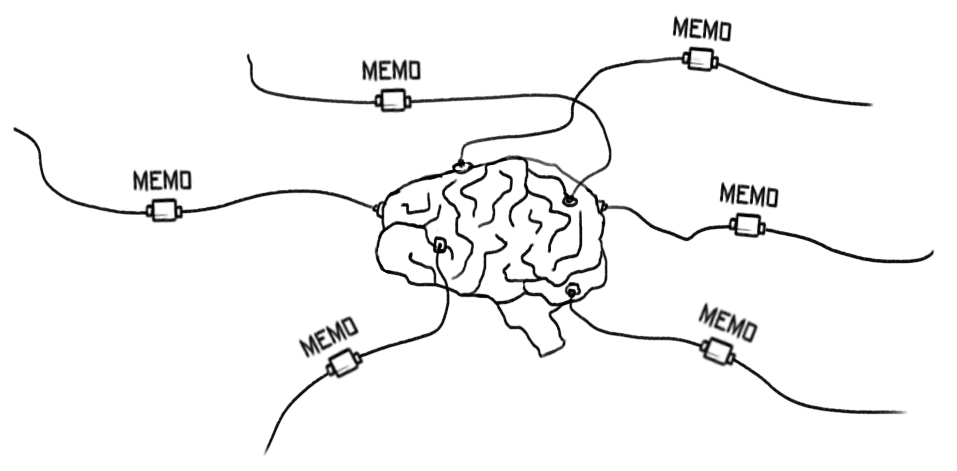# Memoization~ Popular concepts ~The previous chapter showed us that the main instrument in JavaScript is the function. And if something is getting run, again and again, it makes sense to try optimizing it. One way to achieve that is through memoization.

Memoization is capturing and storing the previous execution. Of course, it depends on what the function does, but sometimes we assume that if the arguments are the same, the result will also be the same. In such cases, we could cache the output and return it immediately.

``````function pythagorean(a, b) {
return Math.sqrt(Math.pow(a, 2) + Math.pow(b, 2));
}
``````

The `pythagorean` function does synchronous computation. The result doesn't change if the arguments are the same. It is the perfect candidate for memoization.

``````function pythagorean(a, b) {
console.log('Doing the job ...');
return Math.sqrt(Math.pow(a, 2) + Math.pow(b, 2));
}
function memo(func) {
var cache = {};
return function () {
var key = JSON.stringify(arguments);
if (cache[key]) {
return cache[key];
} else {
return (cache[key] = func.apply(null, arguments));
}
};
}

const mPythagorean = memo(pythagorean);

console.log(mPythagorean(4, 3)); // "Doing the job ..." followed by "5"
console.log(mPythagorean(4, 3)); // only "5"
console.log(mPythagorean(4, 3)); // only "5"
console.log(mPythagorean(4, 3)); // only "5"
``````

Only the first call of `mPythagorean` does the actual work. The rest calls are cheap because `memo` returns the cached result.